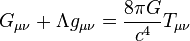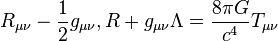# Einstein field equations facts for kids

Kids Encyclopedia Facts

General relativity$G_{\mu \nu} + \Lambda g_{\mu \nu}= {8\pi G\over c^4} T_{\mu \nu}$
Einstein field equations

The Einstein field equations, or Einstein-Hilbert equations, or simply Einstein equations are equations that describe gravity in the classical sense. They are named after Albert Einstein and David Hilbert. The basic idea is to use geometry to model the effects of gravity. The usual form of the equations is that of nonlinear partial differential equations. Such equations are usually solved by approximation. An exact solution can be obtained in special cases, where certain assumptions are dropped, or simplified.

## Mathematical Interpretation

Einstein used mathematical objects called tensors to describe the curvature of spacetime to define gravity. The equation below is the general form of the EFE  :$R_{\mu\nu}-{1 \over 2}g_{\mu\nu},R+g_{\mu\nu}\Lambda={8\pi G \over c^4}T_{\mu\nu}$

Where Rμv is known as the Ricci curvature tensor, gμv is the metric tensor, R is the scalar curvature, Λ is the cosmological constant, G is the gravitational constant, π is pi, c is the speed of light, and Tμv is called the stress-energy tensor.

## Images for kidsEinstein field equations Facts for Kids. Kiddle Encyclopedia.